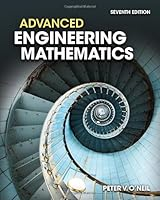# Advanced Engineering Mathematics, 7th Edition29 Reviews
2011-01-01
912 pages

## Book Description

Through previous editions, Peter O'Neil has made rigorous engineering mathematics topics accessible to thousands of students by emphasizing visuals, numerous examples, and interesting mathematical models. Now, ADVANCED ENGINEERING MATHEMATICS features revised examples and problems as well as newly added content that has been fine-tuned throughout to improve the clear flow of ideas. The computer plays a more prominent role than ever in generating computer used to display concepts and problem sets. In this new edition, computational assistance in the form of a self contained Maple Primer has been included to encourage students to make use of such computational tools. The content has been reorganized into six parts and covers a wide spectrum of topics including Ordinary Differential Equations, Vectors and Linear , of Differential Equations and Qualitative Methods, Vector , Fourier , Orthogonal Expansions, and Wavelets, and much more.

PART 1: Ordinary Differential Equations
CHAPTER 1: First-Order Differential Equations
CHAPTER 2: Linear Second-Order Equations
CHAPTER 3: The Laplace Transform
CHAPTER 4: Series Solutions
CHAPTER 5: Approximation of Solutions

PART 2: Vectors, , and Systems of Linear Differential Equations
CHAPTER 6: Vectors and Vector Spaces
CHAPTER 7: Matrices and Linear Systems
CHAPTER 8: Determinants
CHAPTER 9: Eigenvalues, Diagonalization, and Special Matrices
CHAPTER 10: Systems of Linear Differential Equations

PART 3: Vector Analysis
CHAPTER 11: Vector Differential Calculus
CHAPTER 12: Vector Integral Calculus

PART 4: Fourier Analysis, Special , and Eigenfunction Expansions
CHAPTER 13: Fourier Series
CHAPTER 14: The Fourier Integral and Transforms
CHAPTER 15: Special Functions and Eigenfunction Expansions

PART 5: Partial Differential Equations
CHAPTER 16: The Wave Equation
CHAPTER 17: The Heat Equation
CHAPTER 18: The Potential Equation

PART 6: Complex Functions
CHAPTER 19: Complex Numbers and Functions
CHAPTER 20: Complex Integration
CHAPTER 21: Series Representations of Functions
CHAPTER 22: Singularities and the Residue Theorem
CHAPTER 23: Conformal Mappings and Applications

APPENDIX A: A MAPLE Primer

## Book Details

• Title: Advanced Engineering Mathematics, 7th Edition
• Author:
• Length: 912 pages
• Edition: 7
• Language: English
• Publisher:
• Publication Date: 2011-01-01
• ISBN-10: 1111427410
• ISBN-13: 9781111427412